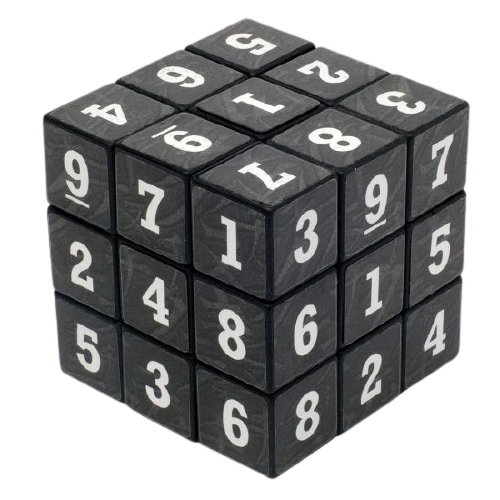# Arithmetic Operatorsarithmetic operators

Arithmetic operators are used in mathematical expressions much the same way they are used in algebra.

Here’s a list of them:

1. Addition (+): Adds the values on either side of the operator.
``````A = 10, B = 5;
A+B = 15``````

2. Subtractions (-): Subtracts values on either side of the operator.

``A-B = 5``

3. Multiplication (*): Multiplies values on either side of the operator.

``A*B = 50``

4. Division (/): Divides the left-hand operand by the right-hand operand.

``A/B = 2``

5. Modulus (%): Returns the remainder of a division operation.

``````A%B = 0
//10 / 5 is 2 with a remainder 0``````

5. Increment (++): Increases the value of the operand by 1

``A++ = 11``

6. Decrement (–): Decreases the value of the operand by 1

``B-- = 4``